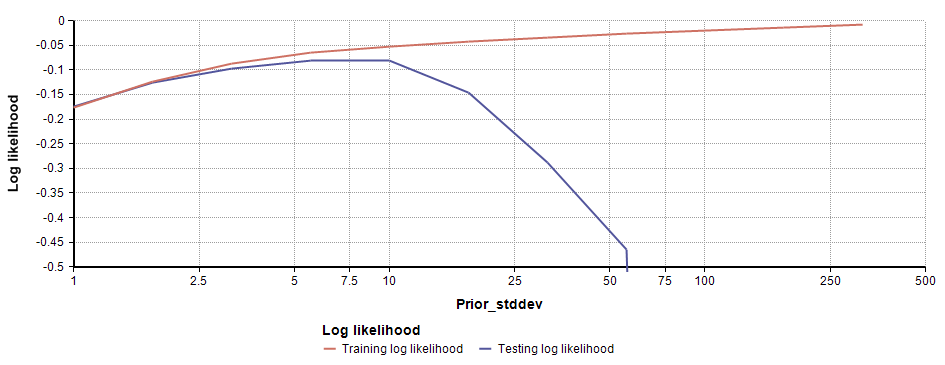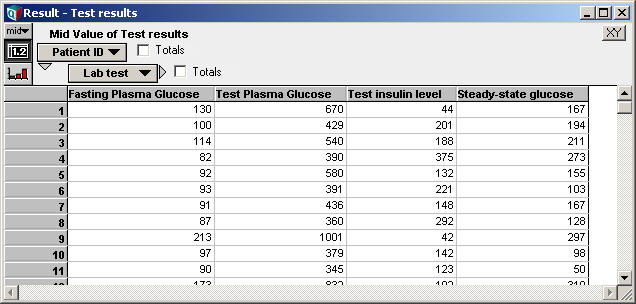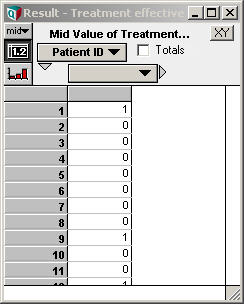# LogisticRegression

Logistic regression is a techique for predicting a Bernoulli (i.e., 0,1-valued) random variable from a set of continuous dependent variables. See the Wikipedia article on Logistic regression for a simple description. Another generalized logistic model that can be used for this purpose is the ProbitRegression model. These differ in functional form, with the logistic regression using a logit] function to link the linear predictor to the predicted probability, while the probit model uses a cumulative normal for the same.

## LogisticRegression(Y, B, I, K, priorType, priorDev)

The LogisticRegression function returns the best-fit coefficients, c, for a model of the form

$\displaystyle{ logit(p_i) = ln\left( {{p_i}\over{1-p_i}} \right) = \sum_k c_k B_{i,k} }$

given a data set basis «B» and classifications of 0 or 1 in «Y». «B» is indexed by «I» and «K», while «Y» is indexed by «I». The fitted model predicts a classification of 1 with probability $\displaystyle{ p_i }$ and 0 with probability $\displaystyle{ 1-p_i }$ for any instance.

The syntax is the same as for the Regression function. The basis may be of a generalized linear form, that is, each term in the basis may be an arbitrary non-linear function of your data; however, the logit of the prediction is a linear combination of these.

Once you have used the LogisticRegression function to compute the coefficients for your model, the predictive model that results returns the probability that a given data point is classified as 1.

## Bayesian Prior

The procedure that fits a logistic regression to data is extremely susceptible to overfititng, and when overfitting occurs, you end up with a model that is too overconfident in its predictions. When logistic regression models overfit the data, they tend to output probabilities very close to 0% and 100% when presented with new data points, when such confidence is unwarranted. This problem is circumvented by using a Bayesian prior, which can also viewed as a penalty function for coefficients.

The «priorType» parameter allows you to select a Bayesian prior. The allowed values are

• 0: Maximum likelihood (i.e., no prior)
• 1: Exponential L1 prior
• 2: Normal L2 prior

The L1 and L2 priors impose a penalty for larger coefficient values, imposing a bias to keep coefficients small. Each imposes a prior probability distribution over the possible coefficient values, independently for each coefficient. The L1 prior takes the shape of an exponential curve, while the L2 prior takes the shape of a normal curve. There is no obvious reason for knowing whether an L1 or L2 would be better for your particular problem, and most likely that choice won't matter much.

The more important component of the prior is the «priorDev», which specifies the standard deviation of the prior -- i.e., how quickly the prior probability falls off. Larger values of «priorDev» correspond to a weaker prior. If you don't specify «priorDev», a guess is made by the function, which will typically be based on very little information. Cross-validation approaches can use the «priorDev» parameter to determine the best prior strength for a problem (see the Logistic Regression prior selection.ana example model in the Data Analysis folder in Analytica for an example).

Weaker priors will almost always result in a better fit on training data (and maximum likelihood should outperform any prior), but on a examples that don't appear in the training set, the performance can be quite a bit different. Typically, performance on new data will improve with weaker priors only up to a point, and then it will degrade and the prior is weakened further. The degradation is from the overfitting phenomena.

These effects are observable in the following log-likelihood graph showing the performance on the training set and on a test set by LogisticRegression with an L2 prior. As you move to the right on the x-axis, the prior is getting weaker (this is the Breast Cancer data set, the graph is from the Logistic Regression prior selection.ana model).## Example

Suppose you want to predict the probability that a particular treatment for diabetes is effective given several lab test results. Data is collected for patients who have undergone the treatment, as follows, where the variable Test_results contains lab test data and Treatment_effective is set to 0 or 1 depending on whether the treatment was effective or not for that patient:Using the data directly as the regression basis, the logistic regression coefficients are computed using:

Variable c := LogisticRegression(Treatment_effective, Test_results, Patient_ID, Lab_test)

We can obtain the predicted probability for each patient in this testing set using:

Variable Prob_Effective := Sigmoid(Sum(c*Test_results, Lab_Test))

If we have lab tests for a new patient, say New_Patient_Tests, in the form of a vector indexed by Lab_Test, we can predict the probability that treatment will be effective using:

Sigmoid(Sum(c*New_patient_tests, Lab_test))

It is often possible to improve the predictions dramatically by including a y-offset term in the linear basis. Using the test data directly as the regression basis requires the linear combination part to pass through the origin. To incorporate the y-offset term, we would add a column to the basis having the constant value 1 across all patient_IDs:

Index K := Concat(, Lab_Test)
Variable B := if K = 1 then 1 else Test_results[Lab_test = K]
Variable C2 := LogisticRegression(Treatment_effectiveness, B, Patient_ID, K)
Variable Prob_Effective2 := Sigmoid(Sum(C2*B, K)

To get a rough idea of the improvement gained by adding the extra y-intercept term to the basis, you can compare the log-likelihood of the training data, e.g.

Ln(Sum(If Treatment_effective then Prob_Effective else 1-Prob_Effective, Patient_ID))

vs.

Ln(Sum(If Treatment_effective then Prob_Effective2 else 1-Prob_Effective2, Patient_ID))

You generally need to use log-likelihood, rather than likelihood, to avoid numeric underflow.

In the example data set that the screenshots are taken from, with 145 patients, the basis without the y-intercept led to a log-likelihood of -29.7, while the basis with the constant 1 produced a log-likelihood of 0 (to the numeric precision of the computer). In the second case the logistic model predicted a probability of 0.0000 or 1.0000 for every patient in the training set, perfectly predicting the treatment effectiveness in every case. On closer inspection I found, surprisingly, that the data was linearly separable, and that the logistic rise had become a near step-function (all coefficients were very large). Although adding the y-offset to the basis in this case led to a substantially better fit to the training data, the result is obviously far less satisfying -- with a new patient, the model will now predict a probability of 0.000 or 1.000 for treatment effectiveness, which is clearly a very poor probability estimate. The phenomena that this example demonstrates is a very common problem encountered in data analysis and machine learning, and is generally referred to as the problem of over-fitting. This example is an extreme case, but it does make it very clear that any degree of overfitting does effectively lead to an "over-confidence" in the predictions from a logistic regression model.

If you are using logistic regression (or any other data fitting procedure for that matter) in a sensitive data analysis task, you should become familiar with the problem of over-fitting, techniques of cross-validation, boot-strapping, etc., to avoid some of these problems.

## History

LogisticRegression is new to Analytica 4.5.

In Analytica 4.4 and earlier, the Logistic_Regression function is available to Analytica Optimizer users in the Generalized Regression.ana library. The built-in LogisticRegression described here is available in all Analytica editions and supersedes the Logistic_Regression function.